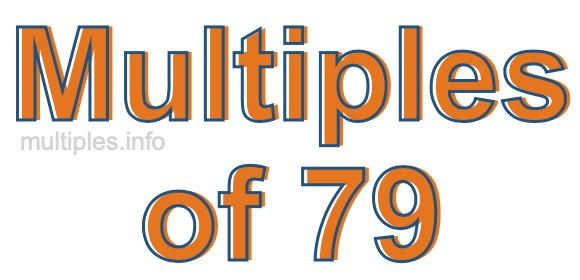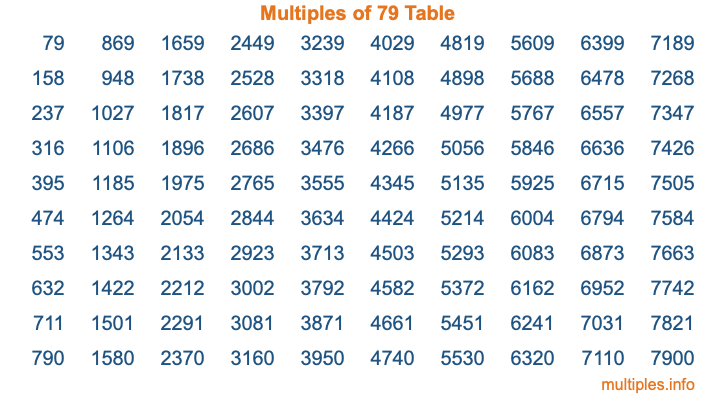Multiples of 79Welcome to the Multiples of 79 page. Here we will first teach you everything you will ever need to know about the multiples of 79, and then give you a study guide summary of everything we taught you to make sure you remember it all. Use this page to look up facts and learn information about the multiples of 79. This page will make you a multiples of seventy-nine expert!

Definition of Multiples of 79
Multiples of 79 are all the numbers that when divided by 79 equal an integer. Each of the multiples of 79 are called a multiple. A multiple of 79 is created by multiplying 79 by an integer.

Therefore, to create a list of multiples of 79, you start with 1 multiplied by 79, then 2 multiplied by 79, then 3 multiplied by 79, and so on for as long as you want. Thus, the list of the first five multiples of 79 is 79, 158, 237, 316, and 395. To see a larger list of multiples of 79, see the printable image of Multiples of 79 further down on this page. We also have a category where you can choose any nth multiple of 79.

Multiples of 79 Checker
The Multiples of 79 Checker below checks to see if any number of your choice is a multiple of 79. In other words, it checks to see if there is any number (integer) that when multiplied by 79 will equal your number. To do that, we divide your number by 79. If the the quotient is an integer, then your number is a multiple of 79.

Is  a multiple of 79?

Least Common Multiple of 79 and ...
A Least Common Multiple (LCM) is the lowest multiple that two or more numbers have in common. This is also called the smallest common multiple or lowest common multiple and is useful to know when you are adding our subtracting fractions. Enter one or more numbers below (79 is already entered) to find the LCM.

Check out our LCM Calculator if you need more details about the Least Common Multiple or if you need the LCM for different numbers for adding and subtraction fractions.

nth Multiple of 79
As we stated above, 79 is the first multiple of 79, 158 is the second multiple of 79, 237 is the third multiple of 79, and so on. Enter a number below to find the nth multiple of 79.

th multiple of 79

Multiples of 79 vs Factors of 79
79 is a multiple of 79 and a factor of 79, but that is where the similarities end. All postive multiples of 79 are 79 or greater than 79. All positive factors of 79 are 79 or less than 79.

Below is the beginning list of multiples of 79 and the factors of 79 so you can compare:

Multiples of 79: 79, 158, 237, 316, 395, etc.

Factors of 79: 1, 79

As you can see, the multiples of 79 are all the numbers that you can divide by 79 to get a whole number. The factors of 79, on the other hand, are all the whole numbers that you can multiply by another whole number to get 79.

It's also interesting to note that if a number (x) is a factor of 79, then 79 will also be a multiple of that number (x).

Multiples of 79 vs Divisors of 79
The divisors of 79 are all the integers that 79 can be divided by evenly. Below is a list of the divisors of 79.

Divisors of 79: 1, 79

The interesting thing to note here is that if you take any multiple of 79 and divide it by a divisor of 79, you will see that the quotient is an integer.

Multiples of 79 Table
Below is an image of the first 100 multiples of 79 in a table. The table is in chronological order, column by column. The first column has the first ten multiples of 79, the second column has the next ten multiples of 79, and so on.The Multiples of 79 Table is also referred to as the 79 Times Table or Times Table of 79. You are welcome to print out our table for your studies.

Negative Multiples of 79
Although not often discussed or needed in math, it is worth mentioning that you can make a list of negative multiples of 79 by multiplying 79 by -1, then by -2, then by -3, and so on, to get the following list of negative multiples of 79:

-79, -158, -237, -316, -395, etc.

Multiples of 79 Summary
Below is a summary of important Multiples of 79 facts that we have discussed on this page. To retain the knowledge on this page, we recommend that you read through the summary and explain to yourself or a study partner why they hold true.

There are an infinite number of multiples of 79.

A multiple of 79 divided by 79 will equal a whole number.

79 divided by a factor of 79 equals a divisor of 79.

The nth multiple of 79 is n times 79.

The largest factor of 79 is equal to the first positive multiple of 79.

79 is a multiple of every factor of 79.

79 is a multiple of 79.

A multiple of 79 divided by a divisor of 79 equals an integer.

79 divided by a divisor of 79 equals a factor of 79.

Any integer times 79 will equal a multiple of 79.

Multiples of a Number
Here you can get the multiples of another number, all with the same attention to detail as we did for multiples of 79 on this page.

Multiples of
Multiples of 80
Did you find our page about multiples of seventy-nine educational? Do you want more knowledge? Check out the multiples of the next number on our list!